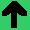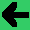# Lab 12: Multi Layer Perceptrons

1. A perceptron takes inputs, sums them, and produces an output based on a transfer function.
2. Make a perceptron with two inputs; use the pass through transfer function. So, it just sums the inputs and outputs the sum. (A linear transfer function with a slope of 1 and no offset is a pass through function).
3. Make weights for the inputs. Replace the sum with the weighted sum and put the weighted sum through the pass through transfer function.
4. Make a linear transfer function, and set the slope to two. Try it with an offset too.
5. Now make two other perceptrons, with the same inputs, but different weights and slopes for the transfer function.
6. Do you see how one perceptron can duplicate the behaviour of 3.
7. Make a step transfer function. This can be used for a linear separator.
8. Now make a different layer of three perceptrons.
9. Make the output from the first layer the input to the second.
10. Weight the connections.
11. Can you make an Xor Categoriser? You may need three layers.
12. You can try to make your own data that is not linearly separable, and make the categoriser categorise all of the data correctly by setting the weights correctly.